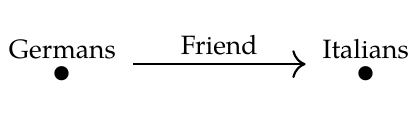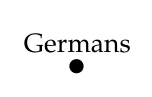By the way, I had things backwards here:

> We're taking \$$\mathcal{C}\$$ to be the free category on this graph

>> and \$$\mathcal{D}\$$ to be the free category on this bigger graph:

>I've fixed it. The lecture now reads:

> We're taking \$$\mathcal{D}\$$ to be the free category on this graph

>> and \$$\mathcal{C}\$$ to be the free category on this bigger graph:

>